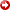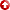--> --> The Relationship Between the Compressional Wave Velocity of Saturated Porous Carbonate Rocks and Density: Theory and Application
[First Hit]# The Relationship Between the Compressional WaveVelocityof Saturated Porous Carbonate Rocks and Density: Theory and Application

## Abstract

Understanding the wavevelocitythrough rocks is essential for the purposes of applied geophysics in such areas as groundwater and hydrocarbon exploration. Theoretically, the wavevelocityis defined by the Newton-Laplace equation. It relates the wavevelocity, V, to the square root of the ratio of the elastic modulus, M, and density, ρ. Therefore, the equation indicates that thevelocityis inversely proportional to density. In-situ field measurements and laboratory experiments of compressional wavevelocitythrough different rocks show otherwise, where thevelocityis directly proportional to approximately the 4th power of density as observed by Gardner. This inconsistency is caused by the interrelationship within the Newton-Laplace equation as the elastic modulus also depends on density. Another observation is the fact that Wyllie’s time average equation also satisfies the 4th power dependence ofvelocityon density. As a result, a new expression for the elastic modulus is derived using Wyllie’s equation and the Newton-Laplace equation. The new equation is a function of the properties of the rock components such as the matrixvelocityand density, fluidvelocityand density, and the bulk density or porosity. This dependence is numerically approximated by , which is obtained using Wyllie’s numerical approximation and the Newton-Laplace equation. In addition, Gardner’s equation is modified to accurately obtain thevelocityover a range of densities (from 1 g/cm3 to around 3 g/cm3). The findings are validated by applying the new expression to field data usingvelocityand density well logs for carbonate rocks as well as the volume fraction of each mineral within the formations. The root mean square error (rmse) between the measuredvelocityand calculatedvelocityis low, which validates the derived expression. The results of this work provide a new model to calculate the elastic modulus for carbonate rocks knowing only the properties of the matrix, the fluid and the bulk density.